#### You may also like### Illusion

A security camera, taking pictures each half a second, films a cyclist going by. In the film, the cyclist appears to go forward while the wheels appear to go backwards. Why?### Weighty Problem

The diagram shows a very heavy kitchen cabinet. It cannot be lifted but it can be pivoted around a corner. The task is to move it, without sliding, in a series of turns about the corners so that it is facing the other way round.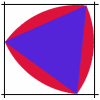### Rolling Triangle

The triangle ABC is equilateral. The arc AB has centre C, the arc BC has centre A and the arc CA has centre B. Explain how and why this shape can roll along between two parallel tracks.

# Attractive Rotations

##### Age 11 to 14Challenge Level

Thank you to Sally and Emily from Parrs Wood for sending us pictures of their rotations. Here are their findings.

Sally noticed a pattern with the coordinates of our shape.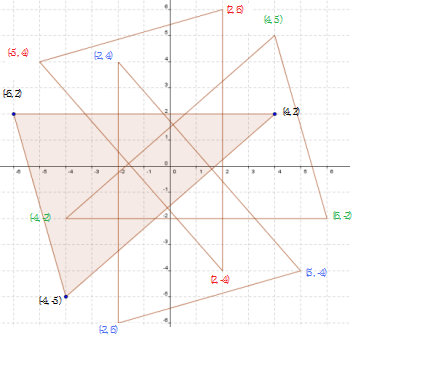In the table below, the coordinates (x',y') describe where the points (x,y) end up after they have rotated by $90^\circ$ around (0,0):

 (x,y) (x',y') (-4,-5) (-5,4) (-6,2) (2,6) (4,2) (2,-4) (-5,4) (4,5) (2,6) (6,-2) (2,-4) (-4,-2) (4,5) (5,-4) (6,-2) (-2,6) (-4,-2) (-2,4) (5,-4) (-4,5) (-2,6) (2,-6) (-2,4) (4,2)

So the coordinates (x',y'), which are a rotation of (x,y) by $90^{\circ}$ around (0,0), are (y, -x).

$180^{\circ}$ rotation is just two $90^{\circ}$ rotations so

(x,y) $\longrightarrow$ (x',y') = (y,-x) $\longrightarrow$ (y',-x') = (-x, -y).
$90^{\circ}$                            $90^{\circ}$

Here are Emily's rotated shapes and mathematical discoveries.

I first rotated shapes by $60^{\circ}$ and found the rotational symmentry was of order 6 because $360\div60 = 6$. But when I coloured them in sometimes the rotational symmentry was of order less than 6. I noticed they were factors of 6.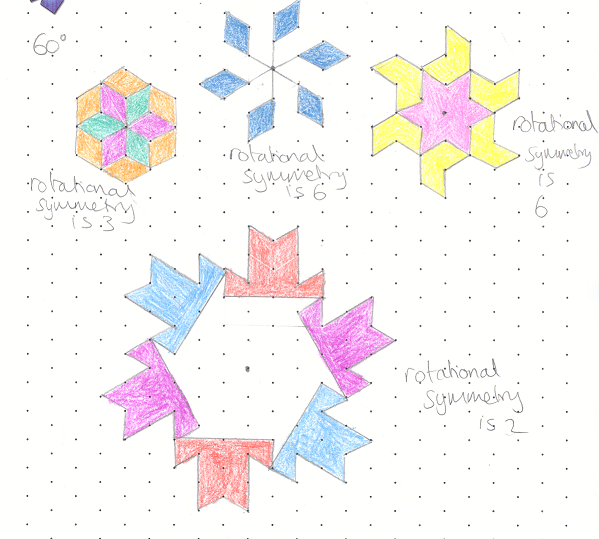I predicted rotations of $30^{\circ}$ would have rotational symmetry of order 12 and $72^{\circ}$ would have rotational symmetry of order 5 because $360\div30 = 12$ and $360\div72 = 5$. These were true for the pictures I drew. I also knew I couldn't colour in my $72^{\circ}$ rotation shape because 5 is prime so its only factors are 5 and 1.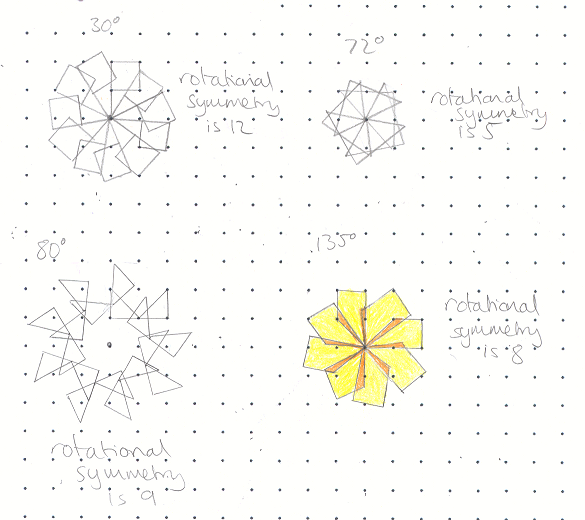$360\div80 = 4.5$ and I had to go round twice to complete my shape. This then gave me rotational symmetry of order 9 because $360\times2=720$ and $720\div80 = 9$.

As $360\div135 = 2{2\over3}$, I worked out $360\times3=1080$ and $1080\div135 = 8$ so I knew I had to go round 3 times to get a shape with rotational symmetry.

Mr Bakker sent us a photo of the work some of his students did on this task: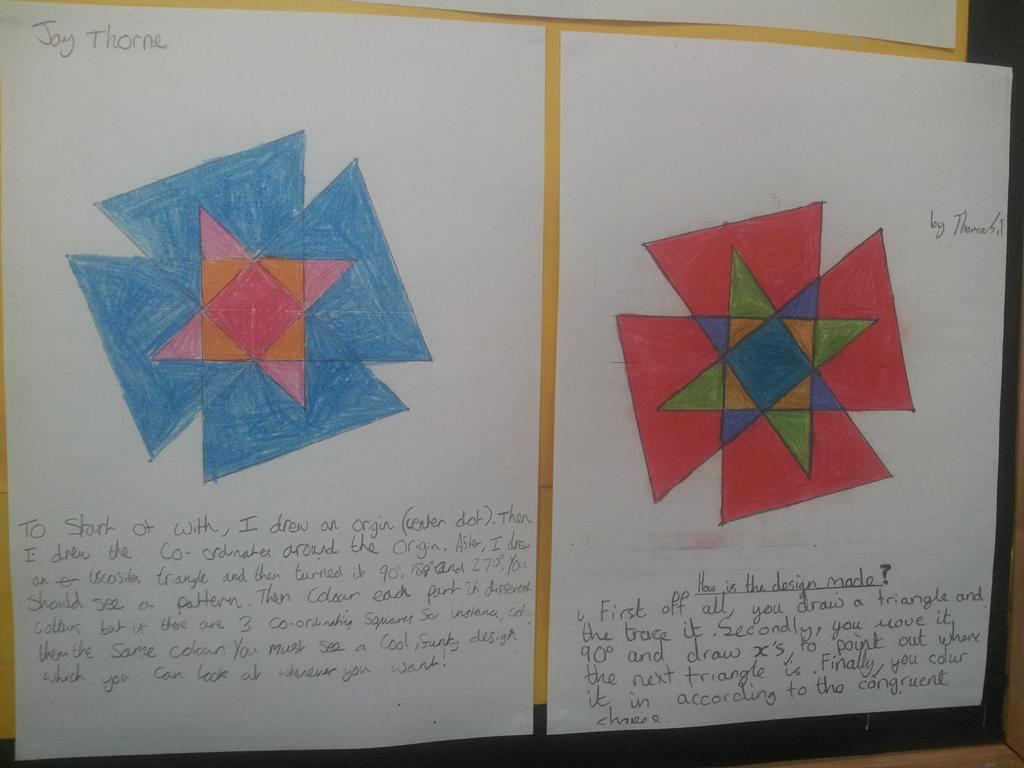Do continue sending us pictures of your rotation patterns along with any interesting mathematical discoveries you make.